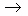Logic
edHelper subscribers - Create a new printable

Number of Keys
 Select the number of different printables: 1 key 2 keys 3 keys 4 keys 5 keys

Number of Pages (for each key)
 Select the number of pages: 1 page 2 pages 3 pages 4 pages

Include an answer key (answer keys will be at the end of the printable)
High School Geometry
High School Geometry
Math

 Name _____________________________Date ___________________
Logic
State the truth value of the following statements.

 1 If p is false and q is false, state the truth for the converse of:~ pq
 2 If p is false and q is true, state the truth for the contrapositive of: p~ q
 3 If p is true and q is true, state the truth for the inverse of:~ p~ q
 4 If p is true and q is false, state the truth for the inverse of: pq
 5 If p is false and q is false, state the truth for the converse of:~ pq
 6 If p is false and q is true, state the truth for the contrapositive of: p~ q
 7 If p is true and q is false, state the truth for the converse of:~ p~ q
 8 If p is true and q is true, state the truth for the inverse of: pq
 9 If p is false and q is true, state the truth for the contrapositive of:~ p~ q
 10 If p is true and q is true, state the truth for the inverse of:~(~ pq )
 11 If p is false and q is false, state the truth for the contrapositive of: p~ q
 12 If p is false and q is true, state the truth for the converse of:~ pq
 13 If p is true and q is false, state the truth for the inverse of:~( pq )
 14 If p is false and q is true, state the truth for the contrapositive of: p~ q
 15 If p is false and q is true, state the truth for the converse of:~ p~ q
 16 If p is false and q is false, state the truth for the converse of:~(~ pq )

Sample
This is only a sample worksheet.

Logic
edHelper subscribers - Create a new printable

Number of Keys
 Select the number of different printables: 1 key 2 keys 3 keys 4 keys 5 keys

Number of Pages (for each key)
 Select the number of pages: 1 page 2 pages 3 pages 4 pages